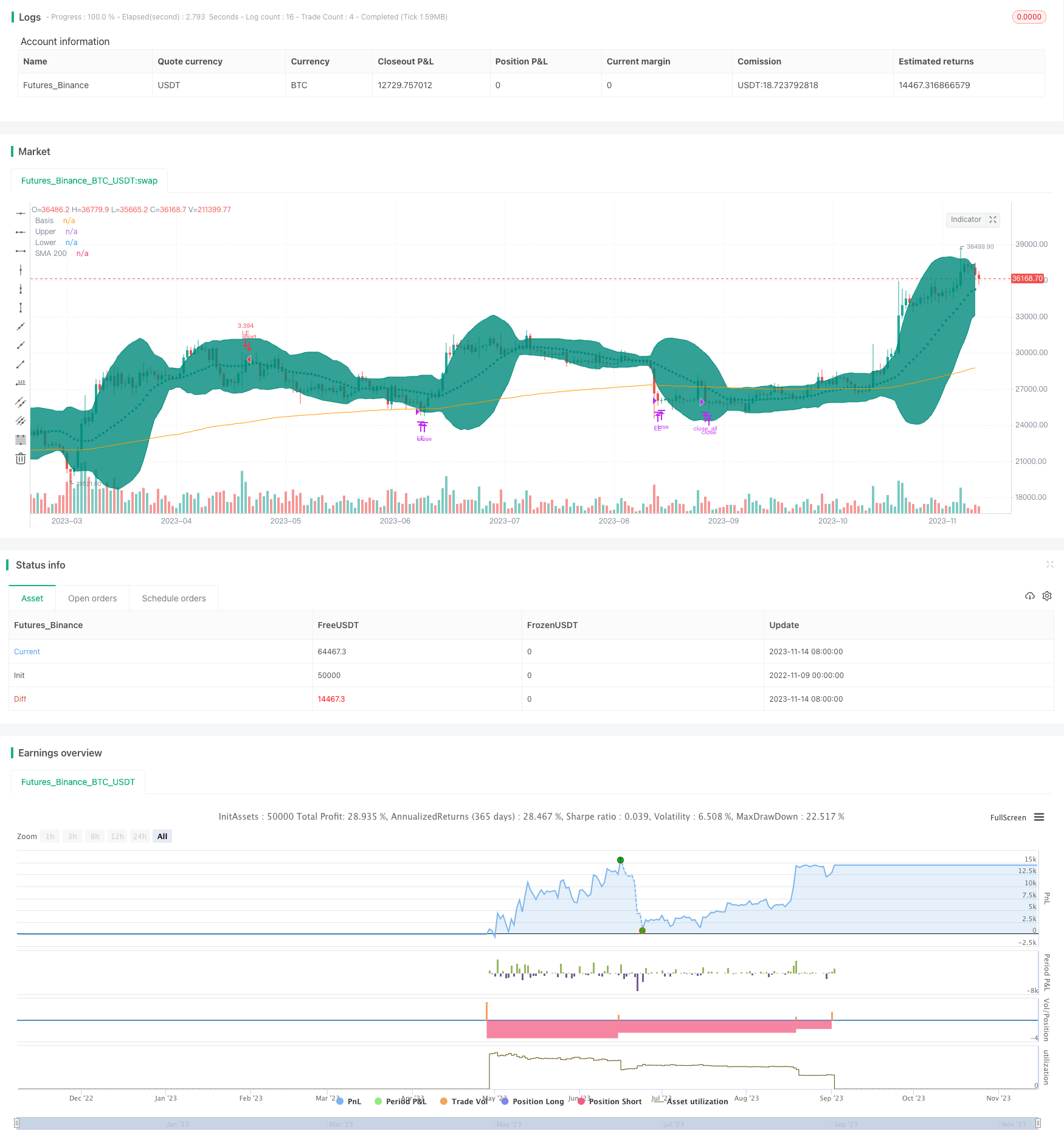# BB21_SMA200 Trend Following Strategy

Author: ChaoZhang, Date: 2023-11-16 11:04:42
Tags:## Overview

This strategy combines Bollinger Bands and Moving Average to design a trend following trading system. It goes long when price breaks through the upper band of Bollinger Bands and the lower band is above SMA200, closes partial position when price breaks through the lower band, and exits all when price crosses below SMA200. The strategy follows the trend and cuts loss in time when trend changes.

## Strategy Logic

1. Calculate SMA200 as Exponential Moving Average to determine the major trend
2. Calculate Bollinger Bands, including upper band, middle band and lower band, and fill color as profit range
3. When both upper and lower bands are above SMA200, it indicates an uptrend
4. When price breaks through the middle band of Bollinger Bands upwards, go long
5. When price breaks through the lower band downwards, close partial position
6. When price crosses below SMA200, it indicates a reversal of the major trend, close all positions
7. Set stop loss point to prevent excessive loss
8. Calculate trade size based on account capital and acceptable risk

The premise of this strategy to identify a trend is that the Bollinger Bands should be completely above SMA200, only going long when a clear uptrend presents. When downtrend comes, risk is controlled by partial stop loss and full stop loss.

1. Use Bollinger Bands instead of a single indicator to identify a clear trend
2. SMA200 determines major trend direction, avoids unnecessary trading in range-bound market
3. Partial stop loss to follow trend runs
4. Timely stop loss on key points to minimize loss
5. Calculate trade size introduces risk management to prevent excessive loss on a single trade

## Risk Analysis

1. Breakout signals generated from Bollinger Bands may have relatively high false signals
2. Partial stop loss points need to be optimized to prevent premature stop loss
3. If stop loss point is too tight, stop loss may be triggered too frequently
4. SMA period needs to be tested and optimized to balance lagging and sensitivity
5. Trade size calculation method may need optimization to prevent excessive size on single trades

These risks could be reduced by carefully testing Bollinger Bands parameters, optimizing partial stop loss strategy, adjusting SMA period, and introducing more scientific risk management methods.

## Optimization Directions

1. Test and optimize Bollinger Bands parameters to lower false signals
2. Research how to set proper partial stop loss points
3. Test optimal SMA period
5. Study using volatility-based position sizing for more scientific trade size calculation
7. Consider combining with other indicators to improve strategy robustness

## Conclusion

This strategy integrates Bollinger Bands and SMA to design a relatively complete trend following system. It is reliable in identifying trend existence and has strong trend tracking capability. By continuously optimizing stop loss strategy, reducing signal errors, and introducing scientific risk management techniques, this strategy can become a worthwhile system to track in live trading. It provides an approach of combining multiple indicators for quantitative trading strategy design.

```/*backtest
start: 2022-11-09 00:00:00
end: 2023-11-15 00:00:00
period: 1d
basePeriod: 1h
exchanges: [{"eid":"Futures_Binance","currency":"BTC_USDT"}]
*/

// This source code is subject to the terms of the Mozilla Public License 2.0 at https://mozilla.org/MPL/2.0/

//@version=4
strategy(title="BB9_MA200_Strategy", overlay=true, pyramiding=1,     default_qty_type=strategy.cash,  initial_capital=10000, currency=currency.USD)  //default_qty_value=10, default_qty_type=strategy.fixed,

var stopLossVal=0.00

//variables BEGIN
smaLength=input(200,title="MA Length")
bbLength=input(21,title="BB Length")

bbsrc = input(close, title="BB Source")
mult = input(2.0, minval=0.001, maxval=50, title="StdDev")

stopLoss = input(title="Stop Loss%", defval=5, minval=1)

riskCapital = input(title="Risk % of capital  == Based on this trade size is claculated    numberOfShares = (AvailableCapital*risk/100) / stopLossPoints", defval=10, minval=1)

sma200=ema(close,smaLength)

plot(sma200, title="SMA 200", color=color.orange)

//bollinger calculation
basis = sma(bbsrc, bbLength)
dev = mult * stdev(bbsrc, bbLength)
upperBand = basis + dev
lowerBand = basis - dev
offset = input(0, "Offset", type = input.integer, minval = -500, maxval = 500)

//plot bb
plot(basis, "Basis", color=color.teal, style=plot.style_circles , offset = offset)
p1 = plot(upperBand, "Upper", color=color.teal, offset = offset)
p2 = plot(lowerBand, "Lower", color=color.teal, offset = offset)
fill(p1, p2, title = "Background", color=color.teal, transp=95)

strategy.initial_capital = 50000

//Entry---

strategy.entry(id="LE", comment="LE capital="+tostring(strategy.initial_capital + strategy.netprofit ,"######.##"), qty=( (strategy.initial_capital + strategy.netprofit ) * riskCapital / 100)/(close*stopLoss/100) , long=true,  when=strategy.position_size<1 and upperBand>sma200 and lowerBand > sma200 and crossover(close, basis) )     //  // aroonOsc<0  //(strategy.initial_capital * 0.10)/close

barcolor(color=strategy.position_size>=1? color.blue: na)

//partial Exit
tpVal=strategy.position_size>1 ? strategy.position_avg_price * (1+(stopLoss/100) ) : 0.00
strategy.close(id="LE", comment="Partial points="+tostring(close - strategy.position_avg_price, "####.##"),  qty_percent=30 , when=abs(strategy.position_size)>=1 and close>tpVal and crossunder(lowerBand, sma200)   )   //close<ema55 and rsi5Val<20 //ema34<ema55

//close All on stop loss
//stoploss
stopLossVal:=   strategy.position_size>1 ? strategy.position_avg_price * (1-(stopLoss/100) ) : 0.00

strategy.close_all( comment="SL Exit points="+tostring(close - strategy.position_avg_price, "####.##"),  when=abs(strategy.position_size)>=1 and close < stopLossVal  )  //close<ema55 and rsi5Val<20 //ema34<ema55  //close<ema89//

strategy.close_all( comment="BB9 X SMA200 points="+tostring(close - strategy.position_avg_price, "####.##"),  when=abs(strategy.position_size)>=1 and  crossunder(basis, sma200)  )  //close<ema55 and rsi5Val<20 //ema34<ema55  //close<ema89

```

More